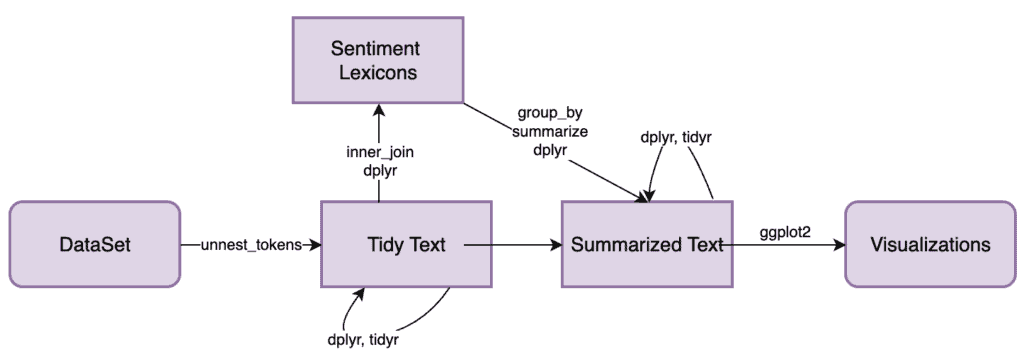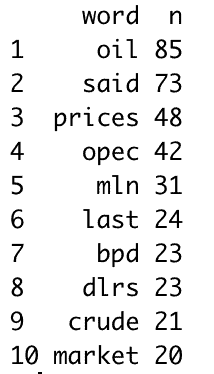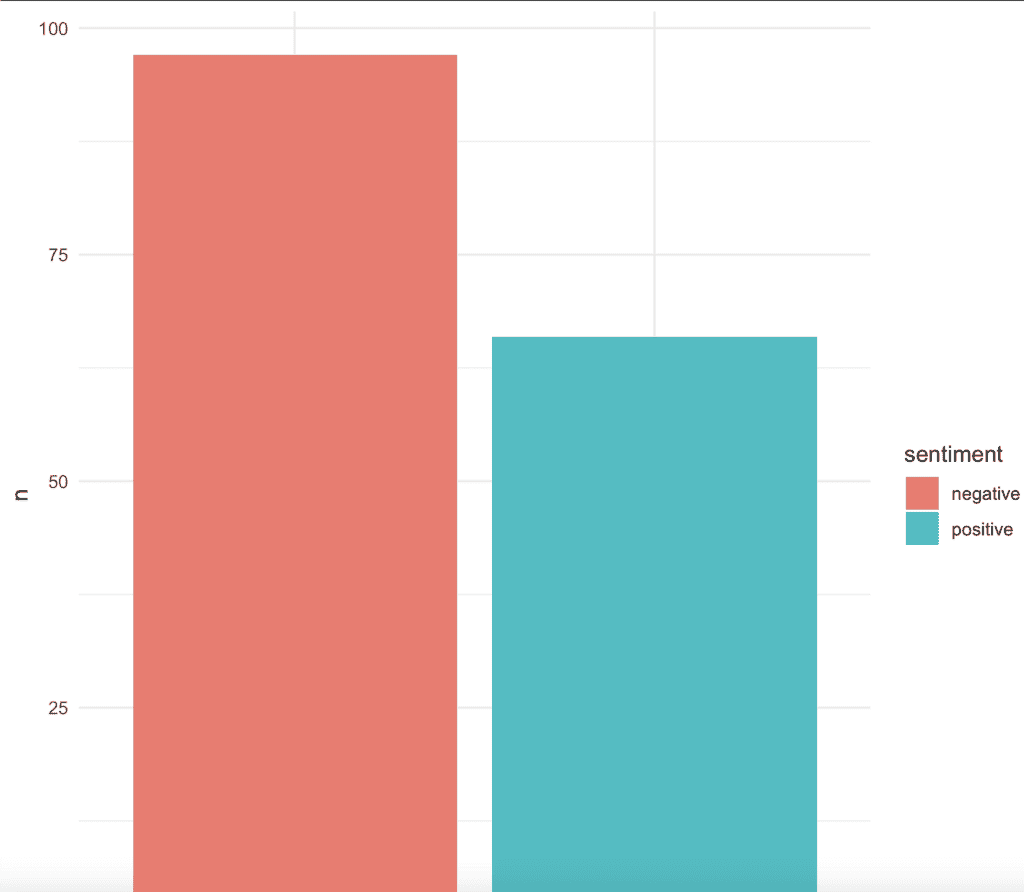# Sentiment Analysis in R: Step-by-Step Project Implementation

Sentiment analysis, often called opinion mining, determines the emotional tone or subjective opinion behind a series of words. It is used to understand the text’s attitudes, emotions, and opinions.

The main objective is to classify the polarity of the text (or its parts), such as positive, negative, neutral, or even more specific emotions like happiness, frustration, sadness, etc.

R provides a rich ecosystem of text mining packages; among them, “tm” and “tidytext” are widely used.

Here is the step-by-step guide to implement a sentiment analysis project with tidy data in R.

## Flow diagram of Sentiment Analysis in R## Step 1: Install the necessary libraries

We need to install three libraries for this project if you have not installed them.

``````install.packages("tidyverse")
install.packages("tidytext")
install.packages("tm")``````

It will install all three packages, and if you have a problem with the “tm” package, you need to upgrade your R to the latest version.

We will use the built-in “crude” dataset in R for a dataset.

You can import the packages and load the data like this:

``````library(tidyverse)
library(tm)
library(tidytext)

data("crude")``````

## Step 2: Data Cleaning & Preprocessing

First, we need to Convert the text to a corpus using the corpus() method.

``````# Convert the text to a corpus
corpus <- crude
``````

Then, preprocess the data.

``````# Preprocess the data
corpus <- tm_map(corpus, content_transformer(tolower))
corpus <- tm_map(corpus, removeNumbers)
corpus <- tm_map(corpus, removePunctuation)
corpus <- tm_map(corpus, removeWords, stopwords("en"))
corpus <- tm_map(corpus, stripWhitespace)``````

Now, convert back to a data frame for tidytext operations.

``````docs_clean <- data.frame(text = unlist(sapply(corpus, `[`, "content")))
``````

## Step 3: Exploratory Data Analysis (EDA)

Exploratory Data Analysis deep dives into three operations.

1. Tokenization
2. Word frequencies
3. Displaying the top 10 words

Let’s explore the most frequently used words.

``````# Tokenization
tokens <- docs_clean %>%
unnest_tokens(word, text)

# Word frequencies
word_freq <- tokens %>%
count(word, sort = TRUE)

# Displaying top 10 words
``````

OutputYou can see that we get this output after running the above code.

## Step 4: Sentiment Analysis

You can analyze sentiments using the “bing lexicon.”

``````# Analyze sentiment
sentiments <- docs_clean %>%
unnest_tokens(word, text) %>%
inner_join(get_sentiments("bing"))

# Count sentiments
sentiment_counts <- sentiments %>%
group_by(sentiment) %>%
tally(sort = TRUE)``````

## Step 5: Visualize the sentiments

You can use the ggplot2 library to visualize the sentiments.

``````# Visualize sentiment distribution
sentiment_plot <- ggplot(sentiment_counts,
aes(x = sentiment, y = n, fill = sentiment)) +
geom_bar(stat = "identity") +
theme_minimal()

sentiment_plot
``````

Output## Conclusion

Based on the visualizations and analysis, you can conclude the sentiments present in the crude dataset.

For example, are they primarily positive or negative? Which words are most commonly associated with positive or negative sentiments?

Remember, sentiment analysis is not always perfect, and results can vary based on the lexicon used and the context of the tweets or reviews.

Always interpret results with caution and consider qualitative insights alongside the quantitative analysis.

You can find the complete code of this project on Github. Copy the code and paste it inside the R Studio, and you will be able to see the output.